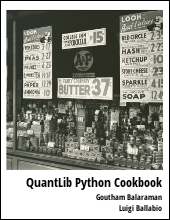Introduces an example on how to value European options using Heston model in Quantlib Python

Visit here for other QuantLib Python examples. If you found these posts useful, please take a minute by providing some feedback.

Heston model can be used to value options by modeling the underlying asset such as the stock of a company. The one major feature of the Heston model is that it inocrporates a stochastic volatility term.

\begin{eqnarray} dS_t &=& \mu S_tdt + \sqrt{V_t} S_t dW_t^1 \\ dV_t &=& \kappa(\theta-V_t) + \sigma \sqrt{V_t} dW_t^2 \end{eqnarray}

Here :

• $S_t$ is the asset's value at time $t$
• $\mu$ is the expected growth rate of the log normal stock value
• $V_t$ is the variance of the asset $S_t$
• $W_t^1$ is the stochastic process governing the $S_t$ process
• $\theta$ is the value of mean reversion for the variance $V_t$
• $\kappa$ is the strengh of mean reversion
• $\sigma$ is the volatility of volatility
• $W_t^2$ is the stochastic process governing the $V_t$ process
• The correlation between $W_t^1$ and $W_t^2$ is $\rho$

In contrast, the Black-Scholes-Merton process assumes that the volatility is constant.

In :
Expand Code

Let us consider a European call option for AAPL with a strike price of \$130 maturing on 15th Jan, 2016. Let the spot price be \$127.62. The volatility of the underlying stock is know to be 20%, and has a dividend yield of 1.63%. We assume a short term risk free rate of 0.1%. Lets value this option as of 8th May, 2015.

In :
Expand Code

Using the above inputs, we construct the European option as shown below.

In :
# construct the European Option
payoff = ql.PlainVanillaPayoff(option_type, strike_price)
exercise = ql.EuropeanExercise(maturity_date)
european_option = ql.VanillaOption(payoff, exercise)


In order to price the option using the Heston model, we first create the Heston process. In order to create the Heston process, we use the parameter values: mean reversion strength kappa = 0.1, the spot variance v0 = volatility*volatility = 0.04, the mean reversion variance theta=v0, volatility of volatility sigma = 0.1 and the correlation between the asset price and its variance is rho = -0.75.

In :
Expand Code

On valuing the option using the Heston model, we get the net present value as:

In :
engine = ql.AnalyticHestonEngine(ql.HestonModel(heston_process),0.01, 1000)
european_option.setPricingEngine(engine)
h_price = european_option.NPV()
print "The Heston model price is",h_price

The Heston model price is 6.5308651372


Performing the same calculation using the Black-Scholes-Merton process, we get:

In :
Expand Code
The Black-Scholes-Merton model price is  6.74927181246


The difference in the price between the two models is: bs_price - h_price = 0.21840667525992163. This difference is due to the stochastic modeling of the volatility as a CIR-process.

# Conclusion

This post provided a minimal example of valuing European options using the Heston model. Comparison with the Black-Scholes-Merton model is shown for instructional purpose.

quantlib   python   finance

Related PostI am Goutham Balaraman, and I explore topics in quantitative finance, programming, and data science. You can follow me @gsbalaraman.

Checkout my bookUpdated posts from this blog and transcripts of Luigi's screencasts on YouTube is compiled into QuantLib Python Cookbook .